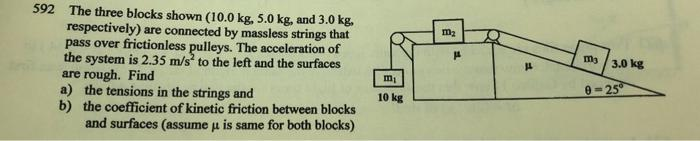# Question Solved1 Answerm2 592 The three blocks shown (10.0 kg, 5.0 kg, and 3.0 kg. respectively) are connected by massless strings that pass over frictionless pulleys. The acceleration of the system is 2.35 m/s to the left and the surfaces are rough. Find a) the tensions in the strings and b) the coefficient of kinetic friction between blocks and surfaces (assume u is same for both blocks) ms 3.0 kg mi 10 kg 0=25Transcribed Image Text: m2 592 The three blocks shown (10.0 kg, 5.0 kg, and 3.0 kg. respectively) are connected by massless strings that pass over frictionless pulleys. The acceleration of the system is 2.35 m/s to the left and the surfaces are rough. Find a) the tensions in the strings and b) the coefficient of kinetic friction between blocks and surfaces (assume u is same for both blocks) ms 3.0 kg mi 10 kg 0=25
More
Transcribed Image Text: m2 592 The three blocks shown (10.0 kg, 5.0 kg, and 3.0 kg. respectively) are connected by massless strings that pass over frictionless pulleys. The acceleration of the system is 2.35 m/s to the left and the surfaces are rough. Find a) the tensions in the strings and b) the coefficient of kinetic friction between blocks and surfaces (assume u is same for both blocks) ms 3.0 kg mi 10 kg 0=25